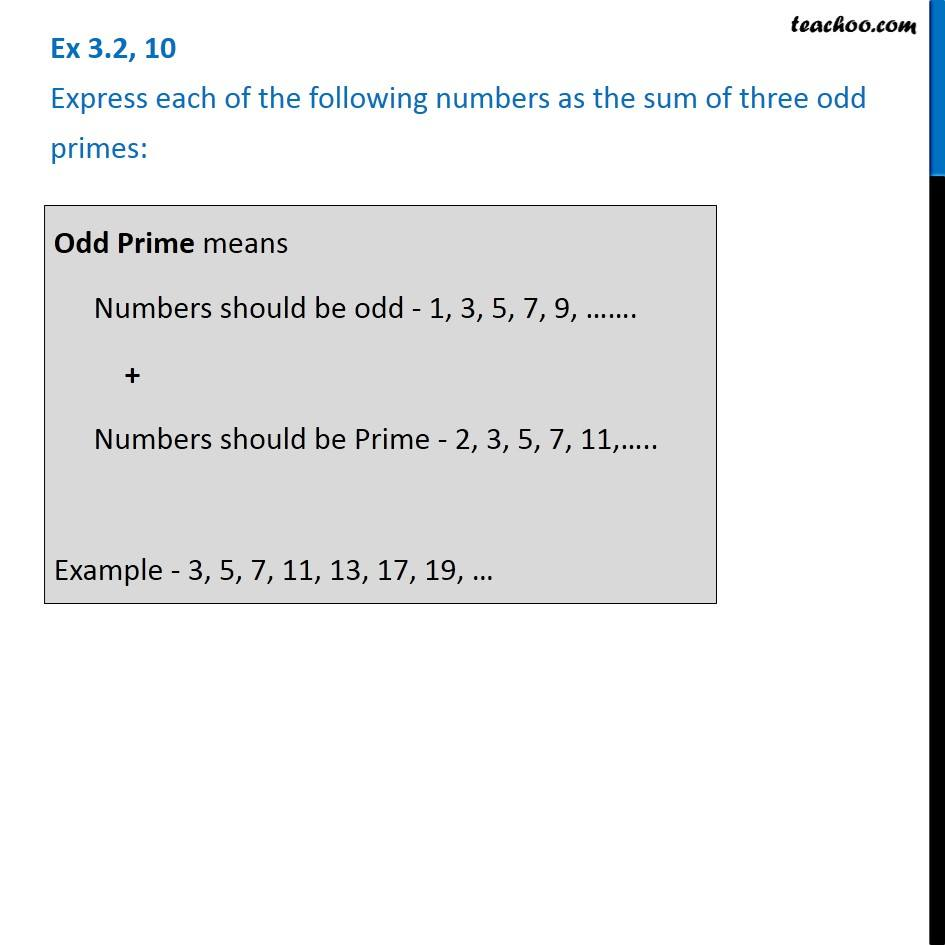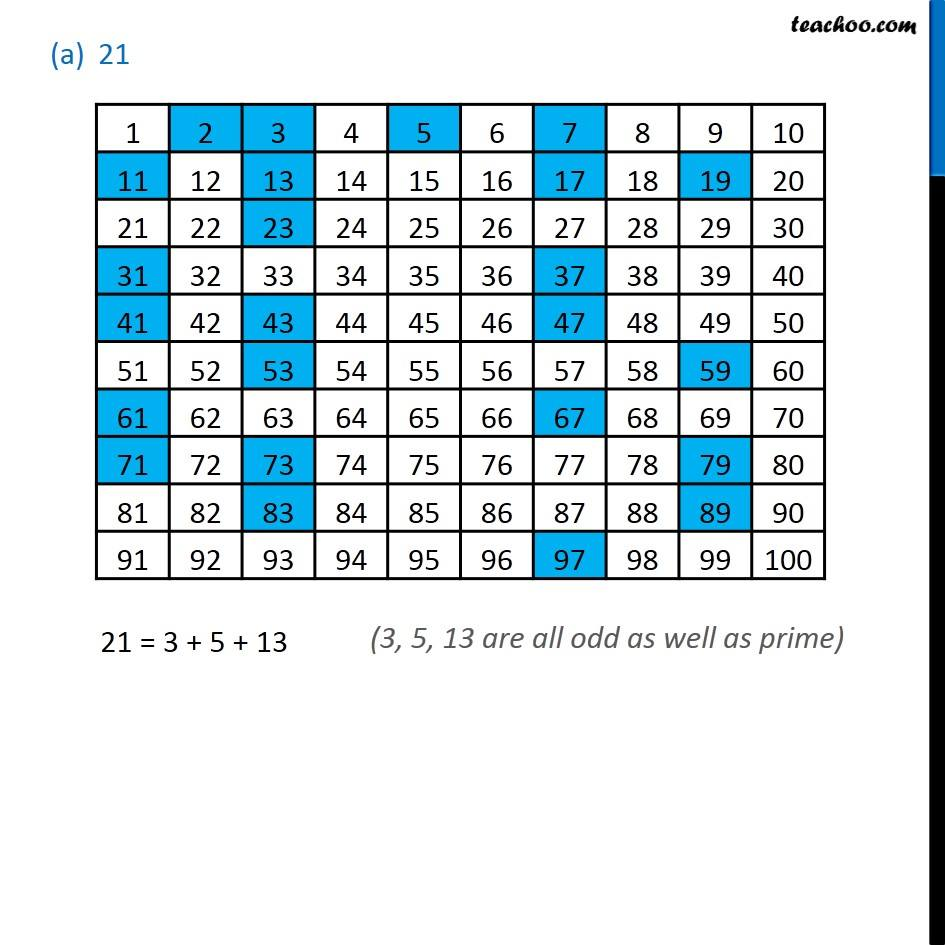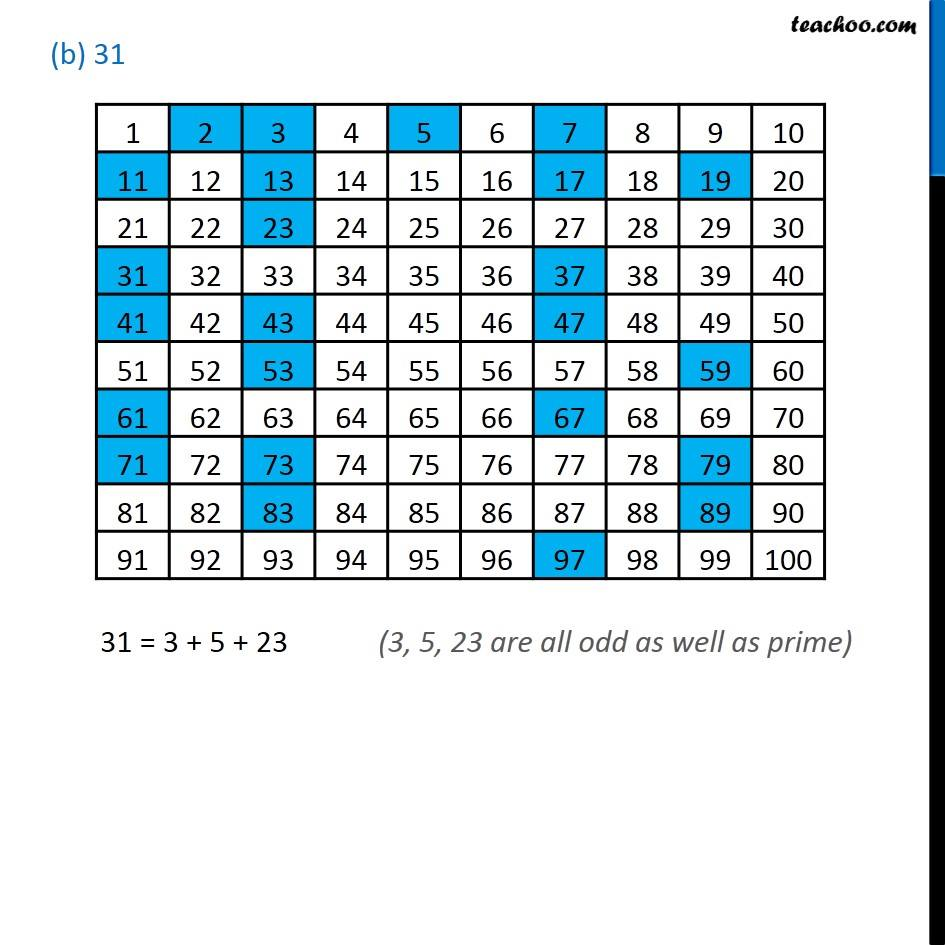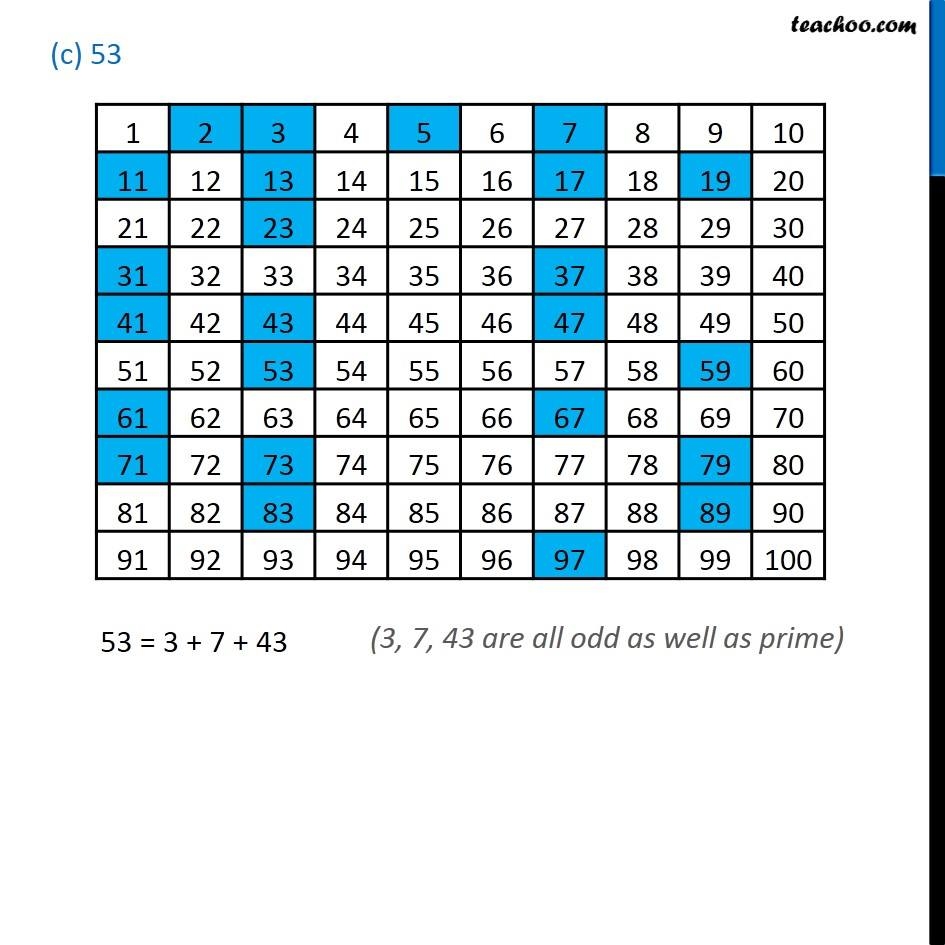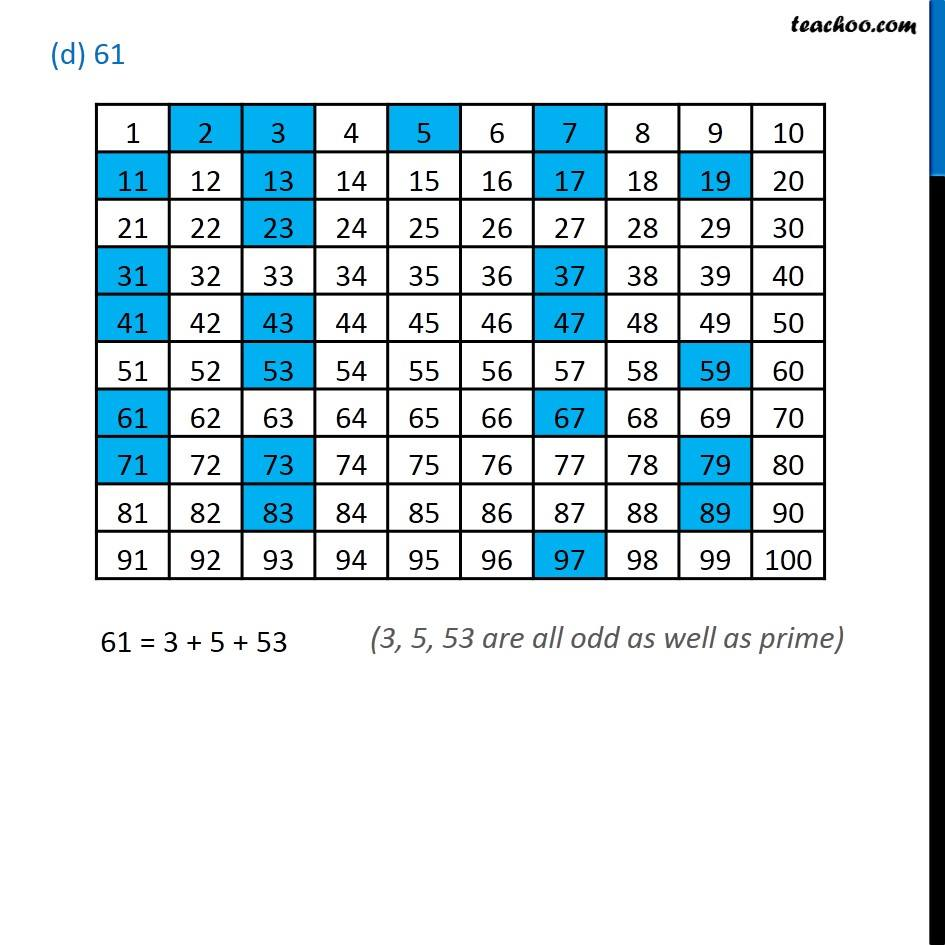1. Chapter 3 Class 6 Playing with Numbers
2. Concept wise
3. Sum of prime numbers

Transcript

Ex 3.2, 10 Express each of the following numbers as the sum of three odd primes: Odd Prime means Numbers should be odd - 1, 3, 5, 7, 9, ……. + Numbers should be Prime - 2, 3, 5, 7, 11,….. Example - 3, 5, 7, 11, 13, 17, 19, … 21 21 = 3 + 5 + 13 (b) 31 31 = 3 + 5 + 23 (3, 5, 23 are all odd as well as prime) (c) 53 53 = 3 + 7 + 43 (3, 7, 43 are all odd as well as prime) (d) 61 61 = 3 + 5 + 53 (3, 5, 53 are all odd as well as prime)

Sum of prime numbers